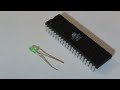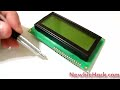### Light Sensitive Resistor (Light Sensor)Introduction:

Do you need to detect if light is present? This will change resistance if the area becomes dark, or light. Use this to detect if night falls, or if a light is turned off/on.

Description:

This is a photoconductive cell, or photo resistor that will change resistance if the light in the area changes.

The resistance will change from 1K to 10K (light to dark respectively). The photodetector can handle 100mW and a max voltage of 150V. Pin spacing is about 3.5 mm.

Light Sensor (Photo Resistor)

\$0.99
Qty:

### Learn about the Light Sensitive Resistor (Light Sensor)Very brief description of a resistor and the resistor's schematic symbol.Physically wiring the circuit for an LED (Light Emitting Diode) to a microcontroller and a resistor so we can turn the LED on and off.Potentiometers are variable resistors. The resistance changes when the wiper is adjusted. The variable resistance is measured from one of the outside leads to the center (wiper) lead. The small vari...The potentiometer is a device that increases or decreases resistance as a variable resistor. Potentiometers can also be used as a voltage divider. If the potentiometer is to be used as a variable res...A voltage divider is a way to decrease a voltage to a proportion of the circuit voltage using two resistors or a potentiometer. The proportion of two resistors will create that proportion output of v... Includes Code